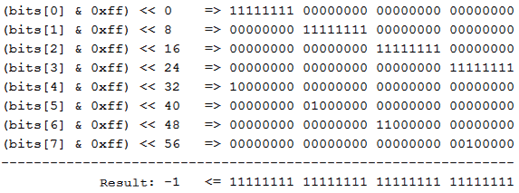﻿ V3134. Shift by N bits is greater than the size of type.# V3134. Shift by N bits is greater than the size of type.

The analyzer detected a likely error that has to do with shifting a value of an integer number by 'N' bits, 'N' being greater than the length of this type in bits.

Consider the following example:

``````UInt32 x = ....;
UInt32 y = ....;
UInt64 result = (x << 32) + y;``````

The programmer intended to form a 64-bit value from two 32-bit ones by shifting 'x' by 32 bits and adding the most significant and the least significant parts. However, as 'x' is a 32-bit value at the moment when the shift operation is performed, shifting by 32 bits will be equivalent to shifting by 0 bits, which will lead to an incorrect result.

This is what the fixed version of the code could look like:

``````UInt32 x = ....;
UInt32 y = ....;
UInt64 result = ((UInt64)x << 32) + y;``````

Now consider the following example from a real project:

``````static long GetLong(byte[] bits)
{
return ((bits & 0xff) << 0)
| ((bits & 0xff) << 8)
| ((bits & 0xff) << 16)
| ((bits & 0xff) << 24)
| ((bits & 0xff) << 32)
| ((bits & 0xff) << 40)
| ((bits & 0xff) << 48)
| ((bits & 0xff) << 56);
}``````

In the 'GetLong' method, an array of bytes is cast to a 64-bit value. Since bitwise shift operations are defined only for 32-bit and 64-bit values, each byte will be implicitly cast to 'Int32'. The bitwise shift range for a 32-bit value is [0..31], so the cast will be performed correctly only for the first 4 bytes of the array.

If the byte array was formed from a 64-bit value (for example 'Int64.MaxValue'), then casting the array back to Int64 using this method will result in an error if the original value was beyond the range [Int32.MinValue....Int32.MaxValue].

For a better understanding of this, let's see what happens when this code is executed over the value '289077008695033855' as an example. When cast to an array of bytes, this value will look as follows:

``289077008695033855 => [255, 255, 255, 255, 1, 2, 3, 4]``

After passing this array to method 'GetLong', each byte will be implicitly cast to Int32 before executing the shift operation. Let's shift each element separately, so we can see where the problem is:As you can see, each shift is performed over a 32-bit value, which causes range overlapping and, therefore, leads to an incorrect result. This happens because when attempting to shift a 32-bit value by more than 32 bits, they are shifted in a circle (shifting by 32, 40, 48, and 56 bits is equivalent to shifting by 0, 8, 16, and 24 bits respectively).

The fixed version of the code above could look like this:

``````static long GetLong(byte[] bits)
{
return ((long)(bits & 0xff) << 0)
| ((long)(bits & 0xff) << 8)
| ((long)(bits & 0xff) << 16)
| ((long)(bits & 0xff) << 24)
| ((long)(bits & 0xff) << 32)
| ((long)(bits & 0xff) << 40)
| ((long)(bits & 0xff) << 48)
| ((long)(bits & 0xff) << 56);
}``````

If we now examine each shift operation separately, we will see that the shifts are performed over 64-bit values, which prevents range overlapping.

 According to Common Weakness Enumeration, potential errors found by using this diagnostic are classified as CWE-128.

410
14 111

### Do you make errors in the code?